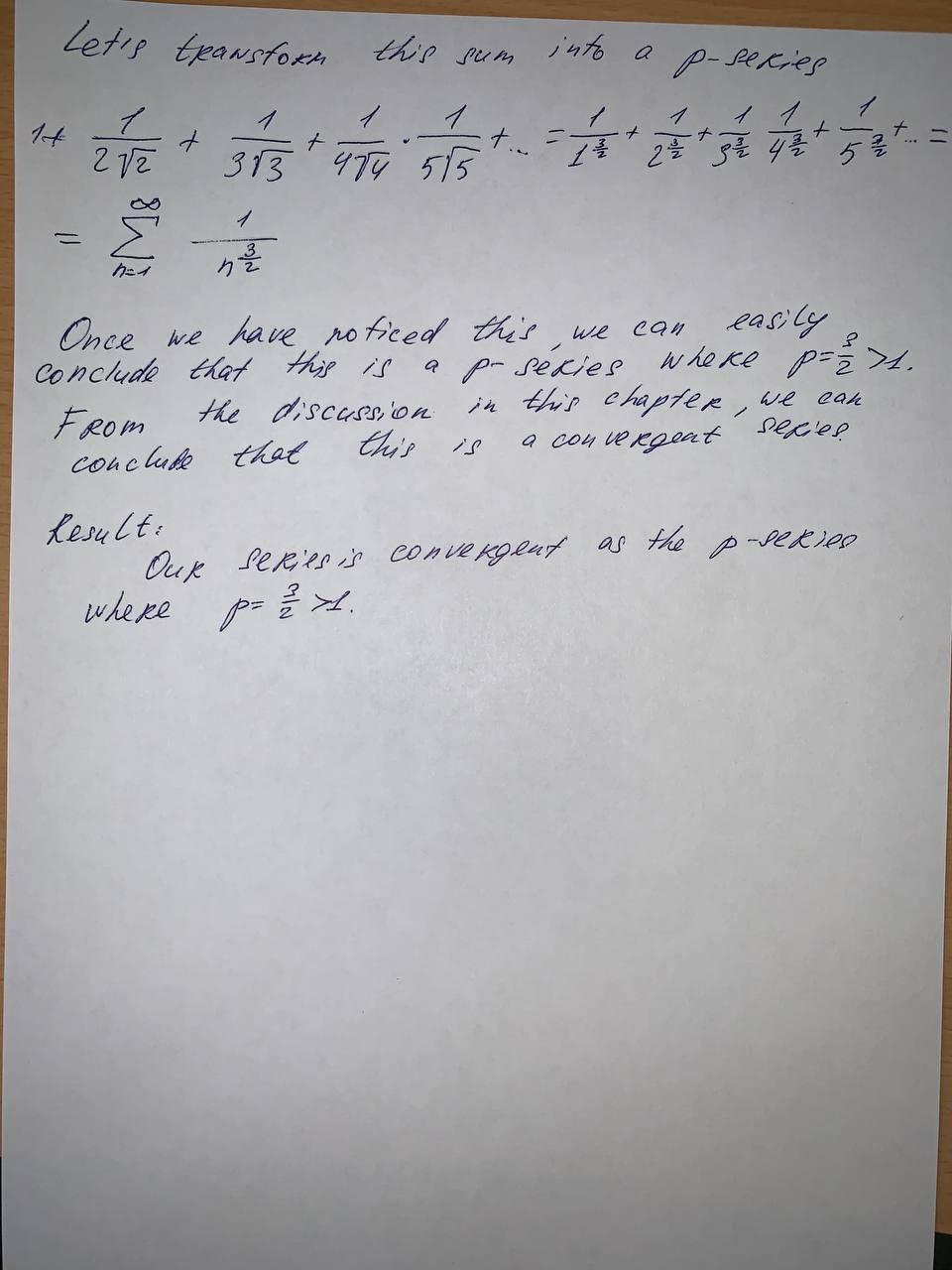Question# Determine whether the series is convergent or divergent.

Series
ANSWEREDDetermine whether the series is convergent or divergent.
$$\displaystyle{1}+{\frac{{{1}}}{{{2}\sqrt{{2}}}}}+{\frac{{{1}}}{{{3}\sqrt{{3}}}}}+{\frac{{{1}}}{{{4}\sqrt{{4}}}}}+{\frac{{{1}}}{{{5}\sqrt{{5}}}}}+\dot{{s}}$$